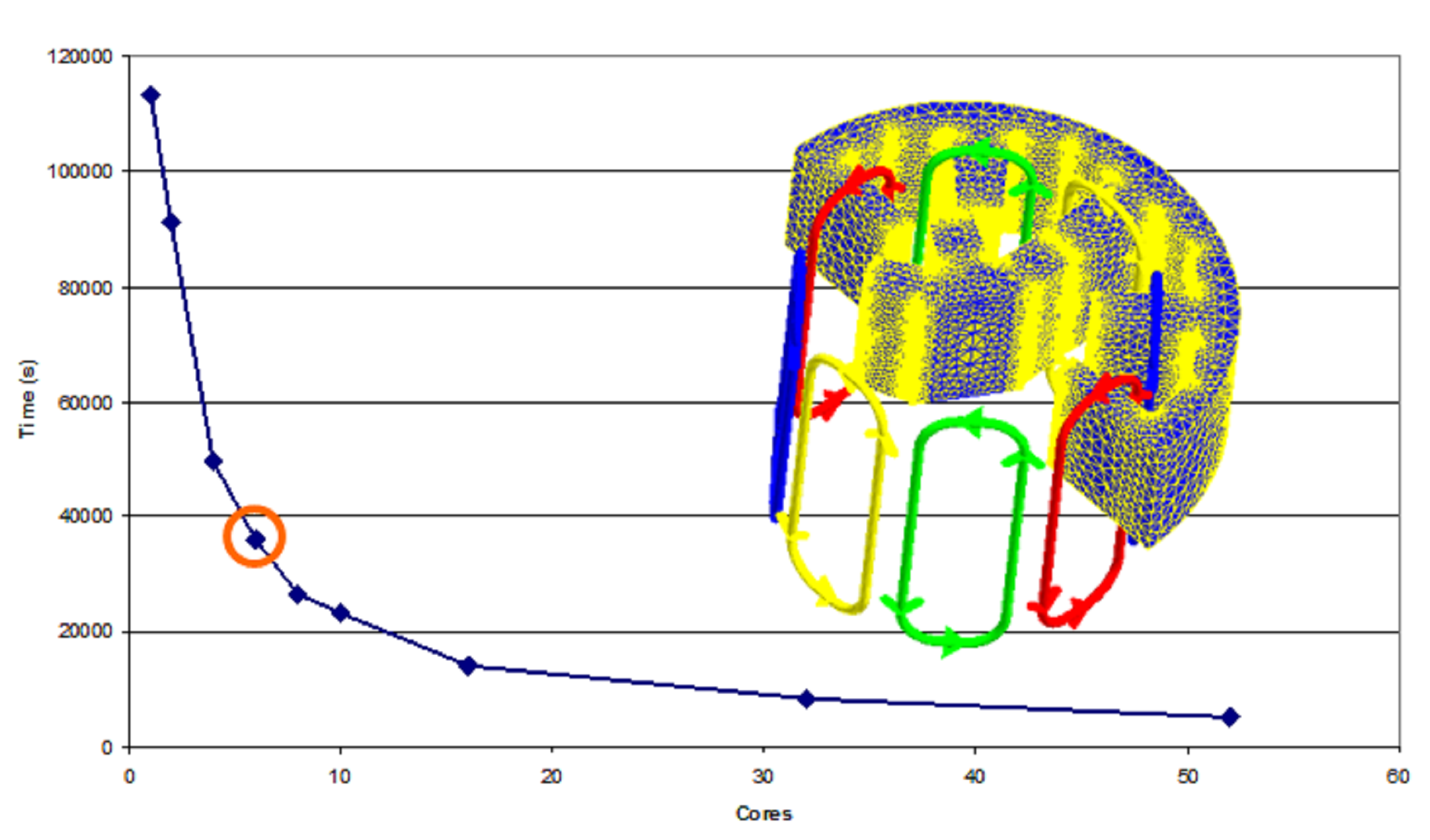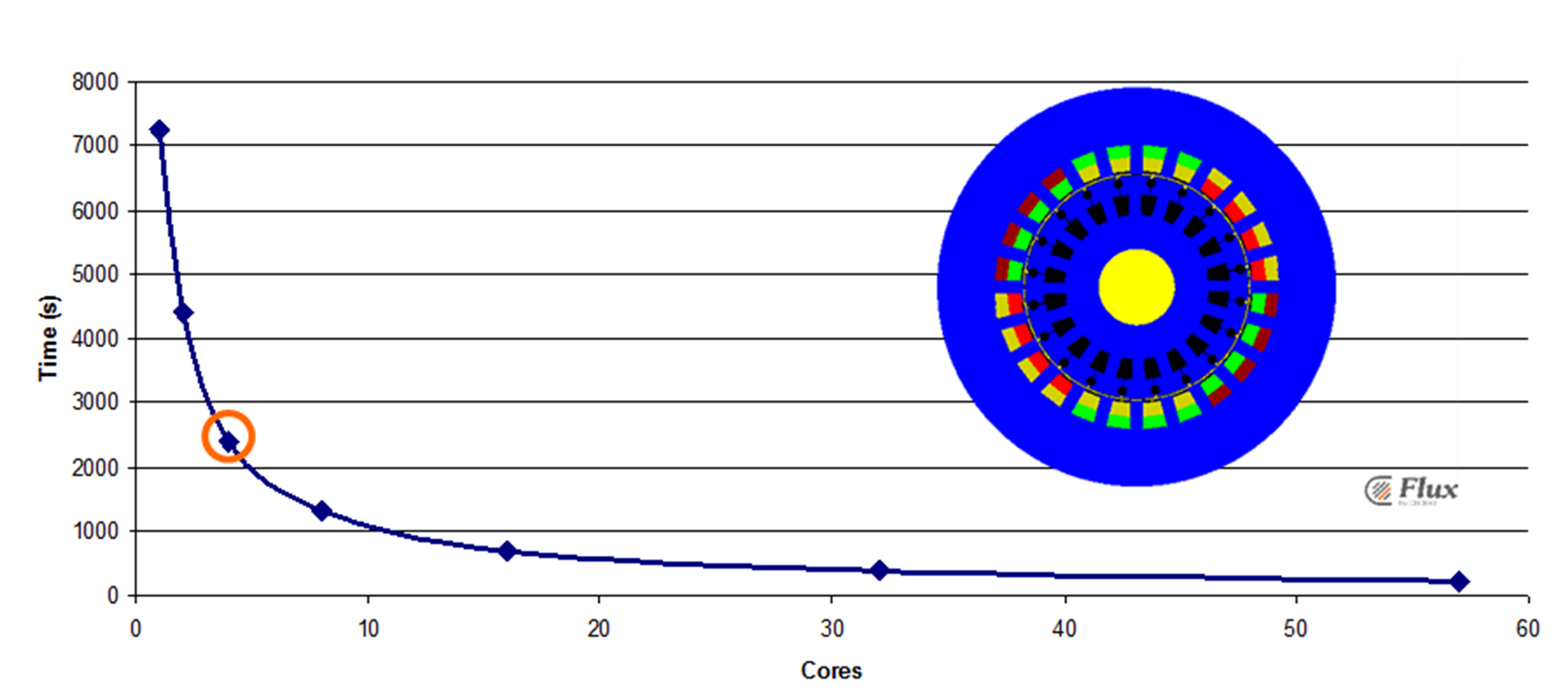# Examples of use

## Introduction

The distributed computing could be used to solve any type of parametric scenario in 2D and 3D varying geometric and physical parameters (except the time).

## 3D switched reluctance motor

The figure below illustrates the computation time reduction for the parametric analysis of an example of 3D switched reluctance motor.In this case by using 6 processors for instance the computation time has been reduced by 3.

## 2D induction motor

The figure below illustrates the computation time reduction for the parametric analysis of an example of 2D induction motor.In this case by using 4 processors for instance the computation time has been reduced by 3.